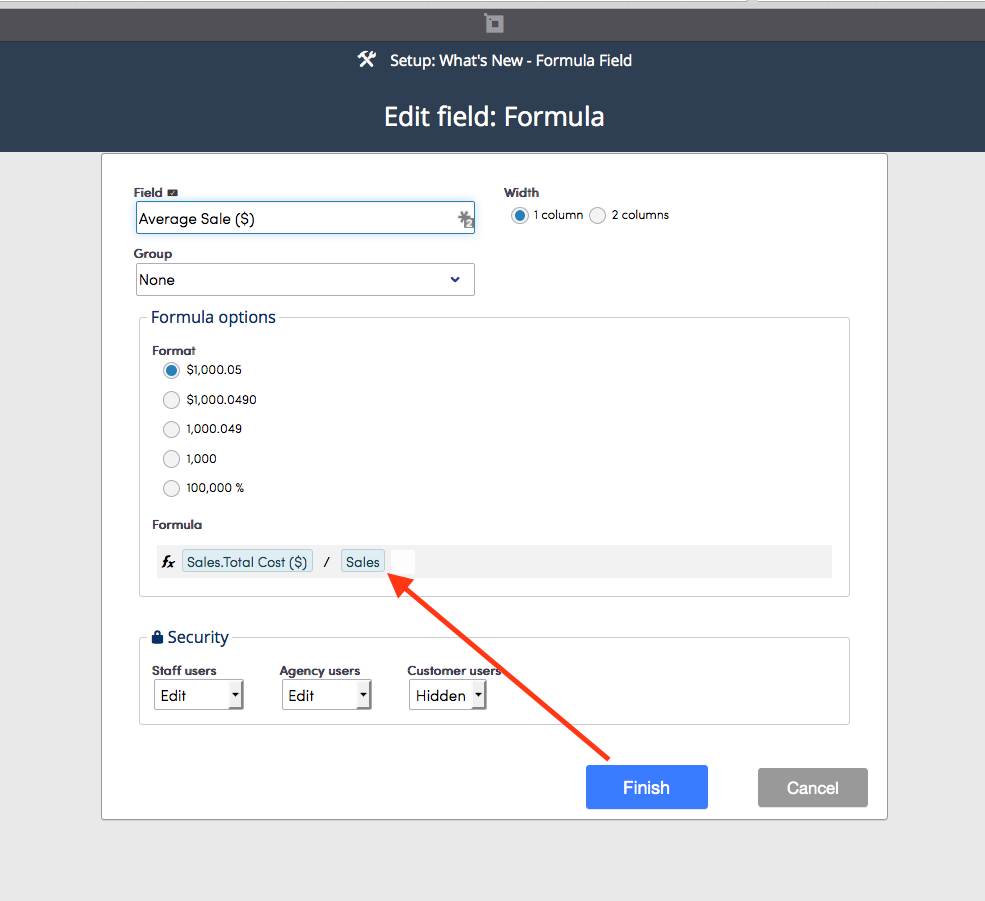Use the formula field to construct formulas using eligible fields in a process.

Consider the form shown in the screenshot below, which displays a simple invoice process that includes a money, quantity, and percent field types. In this process the formula field is used to calculate the Total Cost(\$).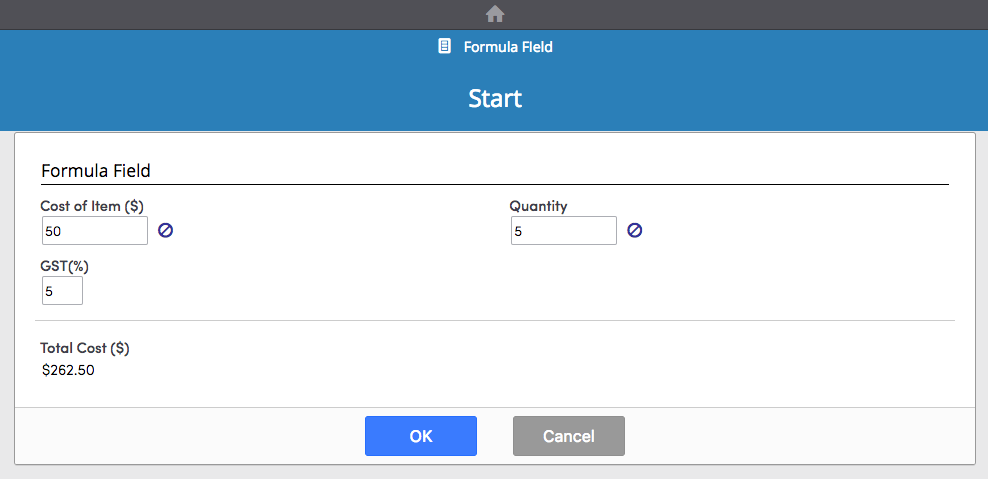To use the formula field, drag the Formula field into the Fields template.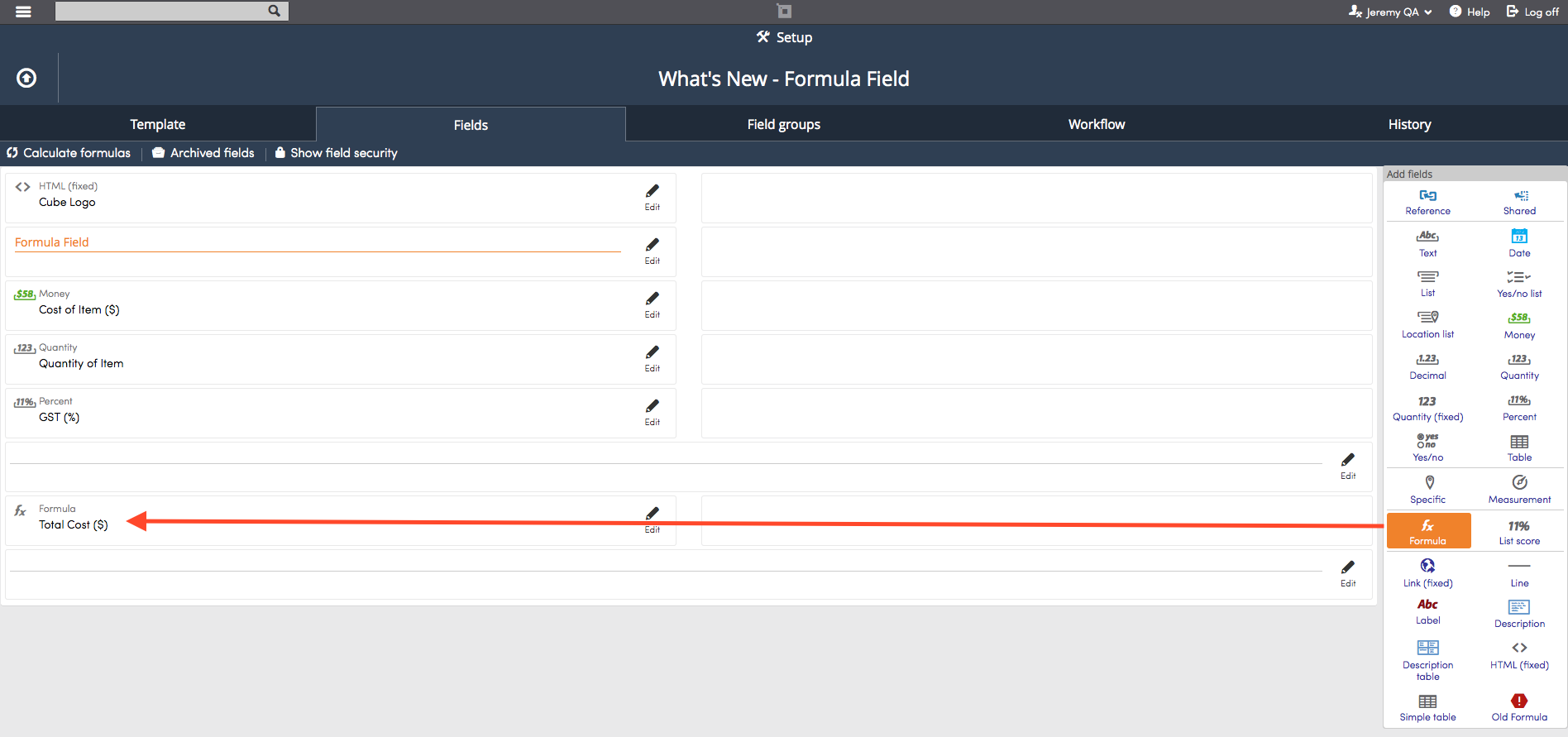Name the Field, choose the Width, and Group.Choose the Format you want the formula's product to display as, either number or date.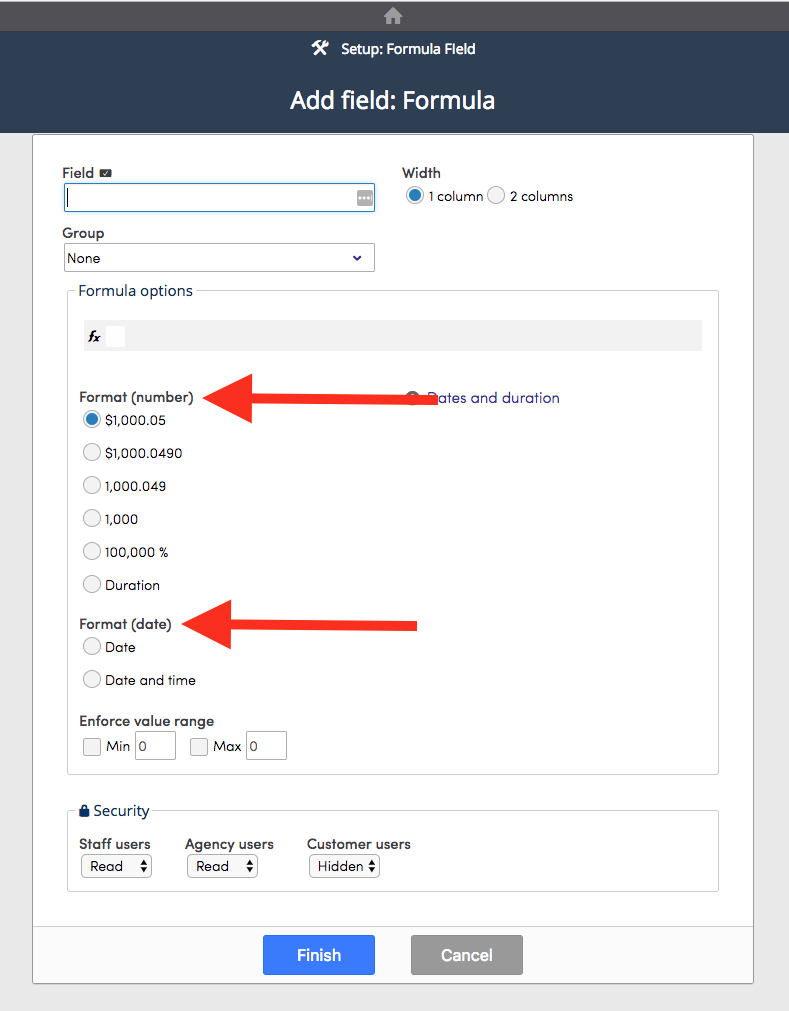The Duration format type will display the Eligible units you can choose to display the duration amount.The Date format type will convert the formula you've created into a date.To create a formula, click the input box beside the fx under Formula. All of the fields in the process will be displayed in a drop down list along with the Operators available to be used to construct a formula.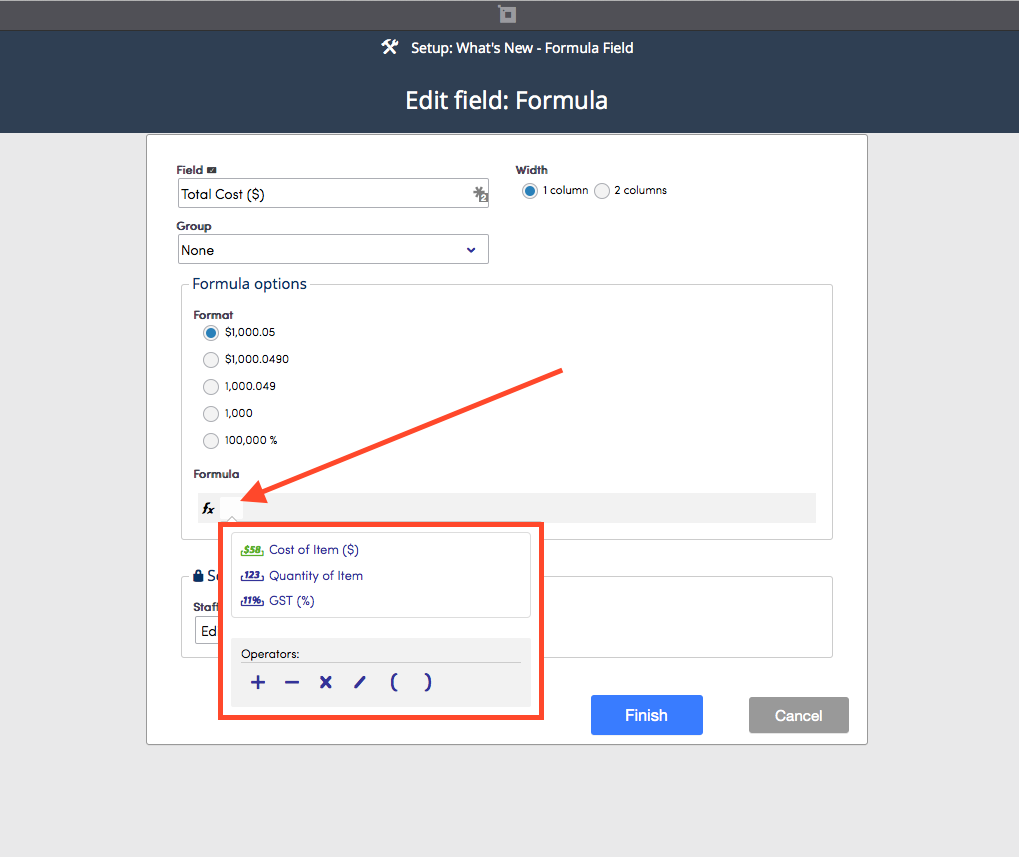Click a field or operator from the drop down list to select it to use in a formula.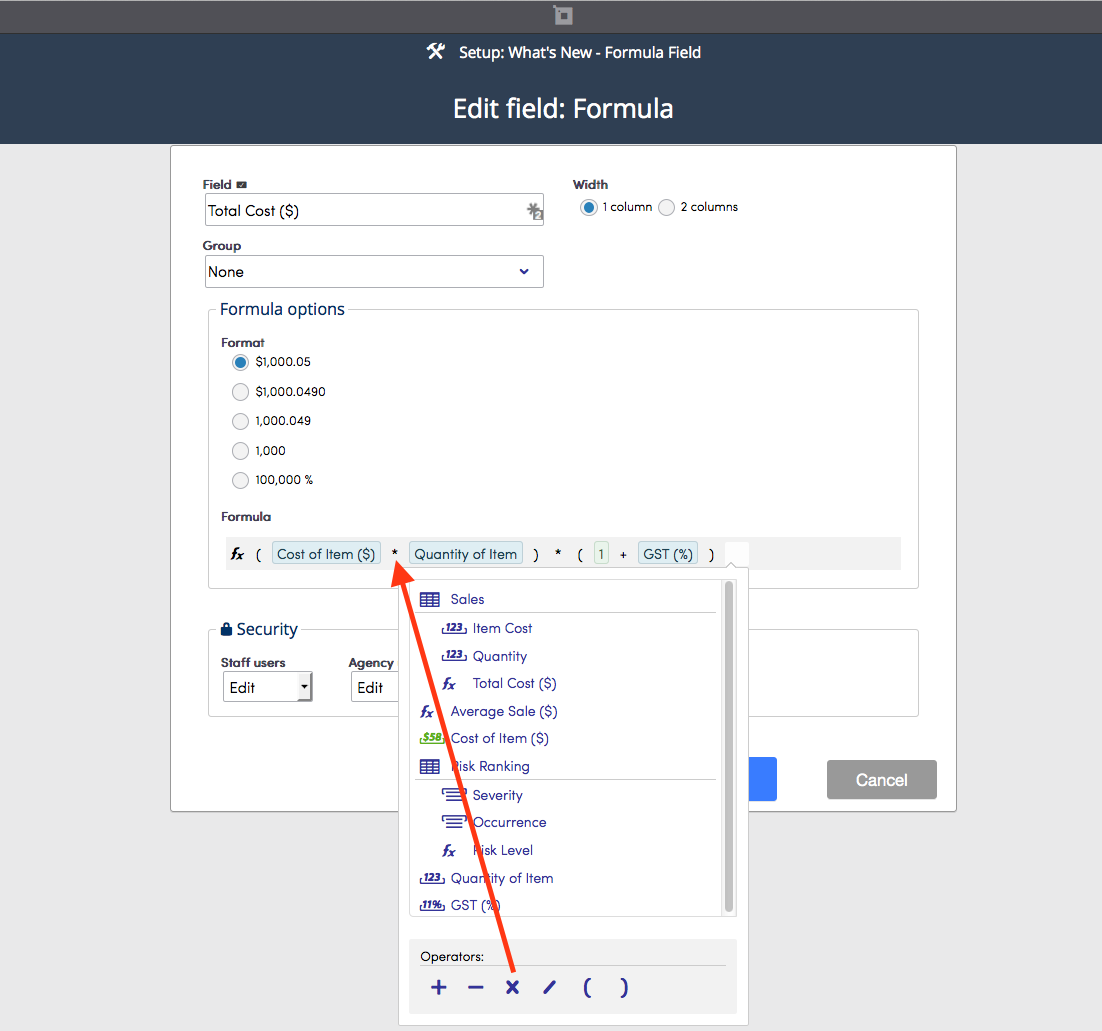If you need to rearrange or insert fields or operators, click and drag them into place. You can also navigate through the formula by using the arrow keys on the keyboard.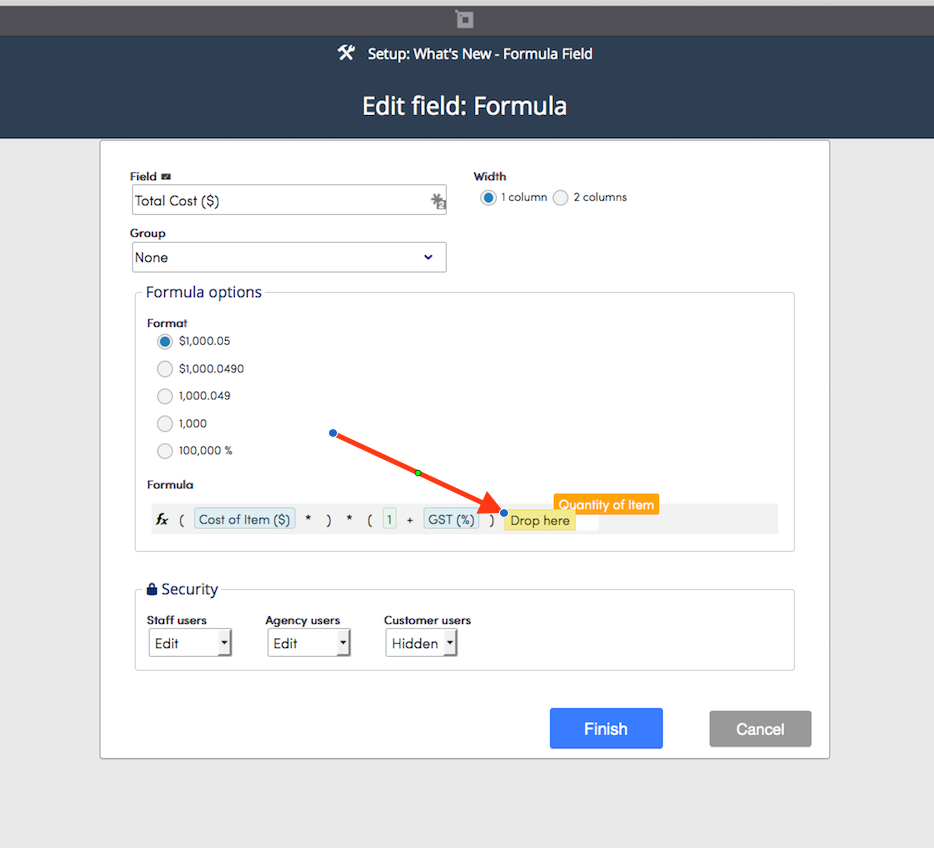To construct the Total Cost (\$) formula used in the invoice example shown in the first screenshot above, brackets must be used. Again, click on the operator to use it in a formula.The Total Cost (\$) formula in the invoice example above also utilized a constant, "1" to calculate GST (a Canadian sales tax). To use a constant in a formula, enter the constant by typing it where appropriate.When the formula is complete, click Finish.### Formula Fields In Table Fields

To use a formula field in a table, click and drag the Formula field into place in the table.Name the field and choose the format you want the formula's product to display as. Add fields to the Formula fx by selecting the field and operators from the drop down list. Fields that belong to a table field will be displayed under the name of the table field. In the screenshot below, the fields that belong to the Risk Ranking table field are highlighted.Adding fields to a formula that belong to a table field will be displayed with the table field's name followed by the field name. For example, notice how the fields in this example are denoted below (Risk Ranking.Severity).### Table Field in Formula Field

The table field itself can be used in the formula field. The table field's value is equal to it's number of rows. Consider the example below. The form calculates the total cost of a list of items and the formula field is used to calculate the average cost of the items in the table.In order to get the average cost of this list, the table field, Sales is used in the formula.